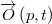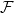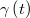﻿

### Geometric characterization of the rotation centers of a particle in a flow

#### Abstract

We provide a geometrical characterization of the instantaneous rotation centers$\overrightarrow{O}\left( p,t\right)$ of a particle in a flow$\mathcal{F}$ over time$t$. Specifically, we will prove that: a) at a specific instant$t$, the point$\overrightarrow{O}\left( p,t\right)$ is the center of curvature at the vertex of the parabola which best fits the path-particle line$\gamma\left( t\right)$ on its Darboux plane at$p$, and b) over time$t$, the geometrical locus of$\overrightarrow{O}\left(p,t\right)$ is the line of striction of the principal normal surface generated by$\gamma\left( t\right)$.

DOI Code: 10.1285/i15900932v36n2p37

Keywords: Geometry of flows; structure of flows

Full Text: PDF